# HESSI Pixon Tutorial

## Introduction

The PIXON method is another technique which removes the sidelobe pattern of a telescope while mitigating the problems of correlated residuals and spurious sources commonly seen in Fourier deconvolution, chi-square fitting, and Maximum Entropy (e.g. Puetter 1995). The Pixon method has been successfully applied to data from the Yohkoh/HXT instrument (Metcalf et al, 1996) and this HXT algorithm has been adapted for use by HESSI.

The PIXON method is similar to the MEM algorithm with the addition of a local condition which defines the spatial scale required by the data at each point in the image. It is also similar to forward fitting in that the Pixon algorithm uses a superposition of elements from a family of multi-resolution basis functions (Pixons) to derive and implement an optimal model with the minimum information content allowed by the data. Hence, the goal of the Pixon method is to construct an image using the simplest possible model while maintaining consistency with the data.

Since the simplest model is used in the reconstruction, the derived image normally has no spurious sources. This has an important implication for the photometry of the image. Within statistical uncertainties, any image reconstructed from HESSI data using any algorithm will have the same total photon flux (summed over the image) as any other valid reconstruction when both fit the data. This is easily demonstrated by a linear superposition of the elements in the reconstruction problem: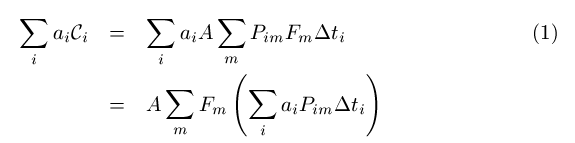where the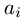are arbitrary constants, P is the modulation patterns, F is the image, and C is the count rate for each modulation pattern. The sum over pixels in the image is represented as a sum of the index m and the sum over time bins is represented as a sum of the index i. Ifcan be found such thatfor all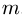, then the total photon flux in any statistically valid image reconstruction is given by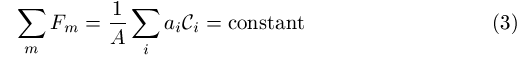For HESSI,can be found which satisfy equation (2) to within a small fraction of a percent. Thus, the total counts in any valid reconstruction must have the same total number of counts (within statistical uncertainties) and it follows that any spurious sources (like sidelobes) in a reconstruction must steal'' counts from real sources. It then follows that the Pixon algorithm will give the best photometry since spurious sources are greatly reduced or eliminated (Alexander and Metcalf, 1997). Note that equation (3) can also be used to calculate the total (unmodulated) flux in an image without doing an image reconstruction.

Although a faster algorithm is possible in principle, the current implementation of the HESSI Pixon algorithm does pay a price for its superior photometry: the method is about an order of magnitude slower than the other reconstruction methods. Therefore, as with Yohkoh/HXT, it will probably be used only after the faster reconstruction algorithms have been used to explore the character of a flare, or when checking the strength or shape of a weak source.

The Figure below shows an example Pixon image reconstruction, and the model source from which simulated countrate profiles were derived. The reconstructed sources are about 1" FWHM, which is the size of the simulated model sources. Even with the logarithmic scaling, no spurious sources are seen.An example of a PIXON map for a simulated double source of 10,000 counts/s/SC. The logarithmic contours show that the dynamic range is over 50:1 in this map.

The current HESSI pixon algorithm uses parabolic pixon basis functions with a circular footprint. The default set of basis functions has diameters given by powers of the square root of two (i.e. 1.00, 1.41, 2.00, 2.83, etc.). The dimensions are user settable, but all basis functions have the circular footprint. It would be possible to expand the basis set to include functions with, for example, an elliptical footprint, but the code would then be hopelessly slow. Any image can be built out of the default pixon basis functions. The only advantage of using a more general set of basis functions would be to reduce the number of degrees of freedom in the reconstruction. However, Pixon reconstructions using the HESSI simulated flare database show that the number of degrees of freedom required to reconstruct typical flares is small so adding more general basis functions would not be worth the added computational expense.

• Alexander, D. and Metcalf, T. R, ApJ, 489, 422, 1997.
• Metcalf, T. R., Hudson, H. S., Kosugi, T., Puetter, R. C., and Pi\~na, R. K., ApJ, 466, 585, 1996. \item
• Puetter, R. C., Int. J. Image Systems Technol., 6, 314, 1995.

## Generating a Pixon Image

### The Command Line Interface

Example call:

iobj = Obj_New('hsi_image',sim_photons=simphotons, $image_dim=[64,64],$
pixel_size=2.0, $sim_energy_band = [ 41., 49.],$
energy_band=[40.,50.])

impixon = iobj->getdata(image_algorithm='pixon',verbose=1)


This call returns then reconstructed image in the array impixon. Other output parameters are available from the "get" method, e.g.

tvscl,iobj->get(/pixonmap)


returns the pixel-by-pixel estimate for the spatial scale of the image. To view all the pixon parameters in a structure, use the command

help,iobj->get(/pixon),/str


Table 1: List of PIXON control parameters:

 pixon_resolution The pixel size of the smallest resolution to search. Using this will not allow any structure at scales less than this pixon size. This is rather restrictive, a more subtle and possibly better way to avoid over-resoltuion is to use the pixon_sensitivity parameter (below). pixon_snr If set to 1, weight the residuals by the signal-to-noise ratio (not very useful). If set to something other than 0 or 1, this parameter does other things for historical reasons, so please do not set it to anything other than 0 or 1. pixon_full_pm_calc If set, do a more complete (but slower) calculation of the pixon map. pixon_background_model If set, attempt a background estimation from the residuals. pixon_variable_metric If set, use the fast algorithm. It is 2 to 4 times faster, but requires a lot of memory. For a 64x64 image, have 300MB or more available. For a 128x128 image, you will likely need 3 to 5 GB. Actually, I don't know how much you need for 128x128, but I do know that my machine does not have enough to test it out! The memory required scales as the fourth power of the linear image dimension. pixon_guess An image used as the initial guess for the pixon reconstruction. The default is to use back projection to compute the initial guess. pixon_sizes A list of pixon sizes (resolutions) to use. The default is powers of sqrt(2.0), e.g. [1.0,1.414,2.0, ...]. Slower, but possibly more robust, would be something like pixon_sizes=findgen(32)+1. pixon_sensitivity The sensitivity used in creating the pixon map. 0.0 is most sensitive, >3.0 is very insensitive. The default is 0.0, i.e. the default is to give the most structure possible in the image. If you get images with structure that you think is spurious, try increasing this parameter to 0.2 or 0.5 to eliminate the over-resolution. pixon_noplot If set, don't do any intermediate plotting. pixon_smpattwritedir If new smoothed patterns are calculated and this keyword is set to a valid directory, then the new smoothed patterns will be saved in that directory. This is very useful (will save you a lot of time) when computing multiple images using a set of parameters not covered in the pixon smoothed pattern database. pixon_progress_bar Set to show the progress bar.

Table 2: List of PIXON output parameters:

 pixon_residual Data residuals. pixon_rgof Final c-statistic. pixon_error An array giving the estimated error on a pixel by pixel basis. OBSOLETE: use error=hsi_calc_image_error(image,object) instead. pixon_iterate The number of iterations used at the final resolution. pixon_outresolution The minimum pixon size used (in pixel units). pixonmap The pixon map giving the spatial scale used at each pixel.

### Intermediate Output

If /verbose is set, the Pixon programs output some interesting information. Here is an example: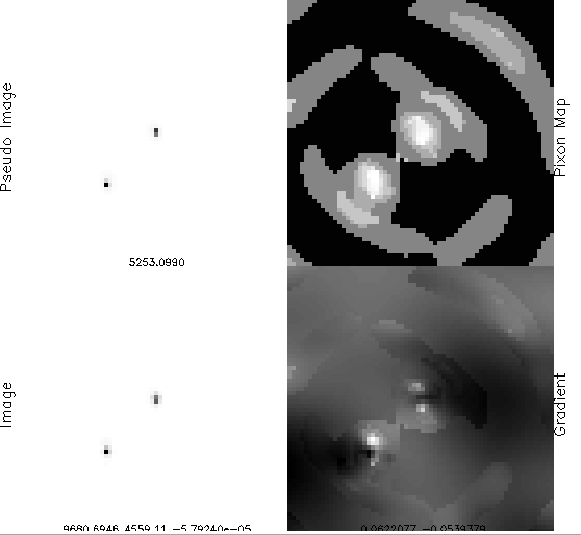The four images show:

 Pseudo Image This shows the pseudo image which is smoothed using the pixon map to generate the current incarnation of the reconstructed image. Image The current image reconstruction. Pixon Map A map showing the current spatial scale being applied at each pixel. This shows you where the information is being concentrated. Gradient The gradient of the goodness-of-fit parameter. This shows where the calculation is going.

## Over-resolution

There are two control parameters that determine the spatial scale of structure the pixon code will search for in the image. The first is pixon_resolution and the second is pixon_sensitivity. Both do more or less the same thing, but from different perspectives.

The pixon_resolution parameter sets a hard limit on the scale of the finest structure allowed in the final image. pixon_resolution is a single floating point number that sets a limit on the pixon sizes which will be used. If you believe that you are seeing spurious structure in the final image, try setting pixon_resolution to 2.0 or 1.4 and see if you are happier with the result. The ratonale for these numbers is that the default pixon sizes (in units of pixels) are [1.0,1.414,2.0,2.828, etc.] (i.e. powers of sqrt(2.0)) and the pixon_resolution control parameter sets the minimum pixon size which is used. So, if you set pixon_resolution to 2.0 then the pixon sizes used are [2.0, 2.828, etc.] and if you set it to 1.4 then the pixon_sizes used are [1.414, 2.0, 2.828, etc.]. These numbers are all in units of pixels, not arc seconds.

The pixon_sensitivity parameter works in a similar fasion, but rather than setting a hard limit on the pixon sizes, the pixon_sensitivity parameter sets the limit based on statistics. The most sensitive setting is 0.0 (the default). If you believe that you are seeing spurious structure in the final image, try setting pixon_sensitivity to 0.2 or 0.5. This number is calibrated to a "sigma" value. If, for example, you set pixon_sensitivity to 1.0, you are asking the code to allow only 1 sigma sources. Note that this statistical limit is set on a pixel by pixel basis. So, 1.0 is actually rather large. For example, if there is a source that covers, say, 100 pixels, then that souce is statistically significant at the 3 sigma level if each pixel has a sigma of 3/sqrt(100)=0.3. So, with pixon_sensitivity set to 1.0, this 100 pixel source would be suppressed even though it is in fact real.

By default, the pixon_resolution and pixon_sensitivity parameters are set to their most sensitive settings (1.0 and 0.0, respectively). The reason for this choice of the defaults is to make sure that no possibly real source is lost in the pixon calculation. But, if you are not happy with the result, then you are free to set the pixon_resolution or the pixon_sensitivity paramters to suit your needs.

## Speed Issues

One of the computationally expensive tasks that the pixon algorithm must do is to smooth all the HESSI modulation patterns using the current pixon map. Thanks to Richard, the modulation patterns are stored in such a way that this calculation can be done once and saved. Hence, to speed up the pixon algorithm, a number of common smoothed modulation patterns are stored in a database.

If you have this SSW database installed on your system, the pixon code will run about twice as fast as it will on a machine without the database installed. But, if you do not have the database, do not despair, it will just take a bit longer to get your pixon image.

To take advantage of the database, use an image size of 32x32, 48x48, or 64x64 with a pixel size of 1"x1", 2"x2", 3"x3" or 4"x4" pixels.

## Background Model

Here is my prescription for modeling the background:
1. Compute the residuals of the modulation profile detector by detector bu subtracting the modulation profile computed from the current image from the observed modulation profile.
2. Phase bin the residuals by spacecraft roll angle into the range [0,2*!pi] to beef up the S/N for cases where the observation includes more than one rotation.
3. For each detector, fit the phase binned residuals to A+B*sin(phi+C)+D*sin(2*phi+E), where phi is the spacecraft roll angle. The B and C terms are often, but not always, small.
4. Undo the phase binning for the function computed in step 3, and set the background to these values.
5. At this stage, if flux_var is set, it is applied to the function computed in step 4. Empirically, this seems to be the right thing to do, though it is not obvious to me that a flux variable background would always be correct.
6. The background is the function arrived at after step 5.
7. In the image iteration, the background is *added* to the modulation profile computed from the image before comparison to the observed modulation profile. (Subtracting the background from the observed modulation profile would mess up the statistics of the fit.)
8. In practice, this procedure rapidly converges to a stable background. What happens in the end is that the image is allowed to soak up any and all counts that can be fit into the modulation. This will include a uniform background over the image in the case of a very extended source. If the residuals based on the image do not look like random noise, then there is assumed to be a background which brings the residuals to the proper statistical form for random noise.

The scheme seems to work quite well, but is OFF by default for Pixons. You can turn it on in the GUI in the "set parameters" menu. For the command line, set bit 3 of pixon_snr, e.g. pixon_snr = pixon_snr OR 4.